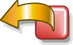## and new primality tests

It is well known (Euler and Lagrange result) that if p=3 [mod 4] is prime, 2p+1 is also prime if and only if 2p+1 divides 2p-1.

I show here that it is possible to find a same result for  p=1 [mod 4], with a new corresponding primality test, a generalization to Cunningham chains of second kind and primality tests of a new type ("in cluster").

#### If p=1 [mod 4] is prime, q=2p+1 is also prime if and only if q divides 2p + 1.

It is also a primality test for Sophie Germain primes with p=4a+1.
It can be used with Proth numbers p=k.2n+1.

Demonstration :
1) If p=1 [mod 4] and q=2p+1 are prime then q divides 2p+1 :
Indeed 22p-1 = (2p-1)*(2p+1)  and 22p-1 = 0 [mod q]  (Fermat th. for q).
As p=1 [mod 4] ,  q=3 [mod 8] , therefore (2/q) = -1 , 2 <> m2 [mod q]  or 2p<> m2p [mod q].
But m2p=1 [mod q] . Therefore q does not divide 2p-1, it divides 2p+1.
2) If p is prime and q=2p+1 divides 2p+1 then q is prime :
Indeed we use the primality test  in n-1 : if for each prime factor f of n-1 there exists an integer a such that
an-1=1 [mod n] and  a(n-1)/f<>1 [mod n] then n is prime.
We have here n-1=2p , 22p= (2p-1)*(2p+1) +1, therefore 22p= 1 [2p+1] since 2p+1 divides 2p+1
22<>1 [2p+1] and 2p=-1<> 1 [mod 2p+1 ] , therefore q=2p+1 is prime.

### Generalization to Cunningham chains of second kind :

#### Theorem 2:

If p=3 [mod 4] and q=2p-1 are prime then q divides 2p-1 + 1.

It is also a pseudo-primality test for Cunningham chains of second kind with p=4a+3 :
If p=3 [mod 4] is prime and q=2p-1 divides 2p-1 + 1,  q is probably prime.

Demonstration :
22p-2-1 = (2p-1-1)*(2p-1+1)  and 22p-2-1 = 0 [mod q]  (Fermat th. for q).
As p=3 [mod 4] ,  q=5 [mod 8] , therefore (2/q) = -1 , 2 <> m2 [mod q]  or 2p-1<> m2p-2 [mod q].
But m2p-2=1 [mod q] . Therefore q does not divide 2p-1-1, it divides 2p-1+1.

#### Theorem 3:

If p=1 [mod 4] and q=2p-1 are  prime then q divides 2p-1 - 1.

It is also a pseudo-primality test for Cunningham chains of second kind with p=4a+1 :
If p=1 [mod 4] is prime and q=2p-1 divides 2p-1 - 1,  q is probably prime.

Demonstration :
22p-2-1 = (2p-1-1)*(2p-1+1)  and 22p-2-1 = 0 [mod q]  (Fermat th. for q).
As p=1 [mod 4] ,  q=1 [mod 8] , therefore (2/q) = 1 , 2 = m2 [mod q]  or 2p-1= m2p-2 [mod q].
But m2p-2=1 [mod q] . Therefore q divides 2p-1-1.

But as p is prime, p divides also 2p-1-1.

#### Theorem 3a :

If p=1 [mod 4] and q=2p-1 are  prime then p*q divides 2p-1 - 1.

It is also a pseudo-primality test for Cunningham chains of second kind with p=4a+1 :
If n=1 [mod 4] and n1=2n-1  and if n*n1 divides 2n-1 - 1,  then n and n1 are probably prime.

The generalization of this test is even evident and immediate, since n=1 [mod 4], 2n-1=1[mod 4]  :
Let pi, pi+1, pi+2 prime such that pi=1[mod 4] , pi+1=2pi-1, pi+2=2pi+1-1.
Therefore we have 2^(pi+1-1)-1=0 [mod pi+1*pi+2], but 2^(pi+1-1)-1=2^(2(pi-1))-1 and 2^(pi-1)-1=0[mod pi],
2^(pi+1-1)-1=0 [mod pi*pi+1*pi+2].
Therefore this relationship is necessary in order that pi, pi+1, pi+2 are prime.

Pseudo test "in cluster" :

If n=1 [mod 4] and n1=2n-1,  n2=2n1-1, ..., ni=2ni-1-1, and if n*n1*n2*..*ni divides 2^(ni-1-1) - 1,
then  n,n1,n2, .., n are probably prime, otherwise one at least of numbers n,n1,n2, .., ni is composite.
This method that allows a pseudo test of several numbers at the same time can speed up considerably the research of Cunningham chains of second kind with any length. It is even well adapted to test numbers Proth n=k.2n+1 (form 4a+1) "in cluster", a complete test of each number being undertaken only if this first test passes.

In summary :

 p prime 2p+1 prime ? 2p-1 prime ? p=1 [mod 4] New test  2p+1 = 0 [mod 2p+1] New probable test 2p-1-1 = 0 [mod 2p-1] p=3 [mod 4] Euler-Lagrange test 2p-1 = 0 [mod 2p+1] New probable test 2p-1+1 = 0 [mod 2p-1]

 n=1 [mod 4]  n1=2n-1,  n2=2n1-1, ..., ni=2ni-1-1 New probable test "in cluster"   2^(ni-1-1) - 1 = 0 [mod n*n1*n2*..*ni]

Complements to these tests (soon ...)

You can also consult the next links :

Created by  Henri Lifchitz : November , 5  1998, last modification: November, 20  1998.